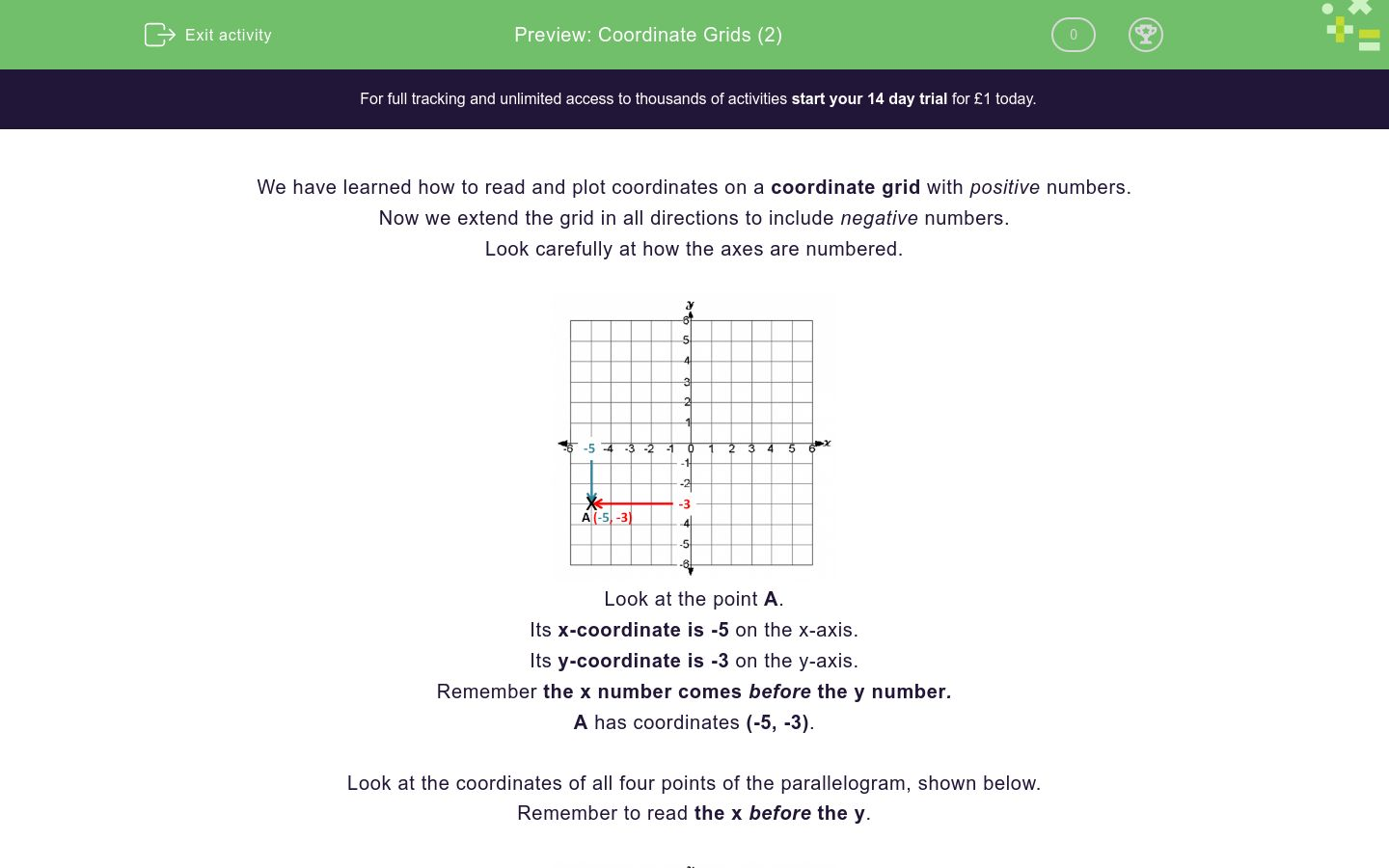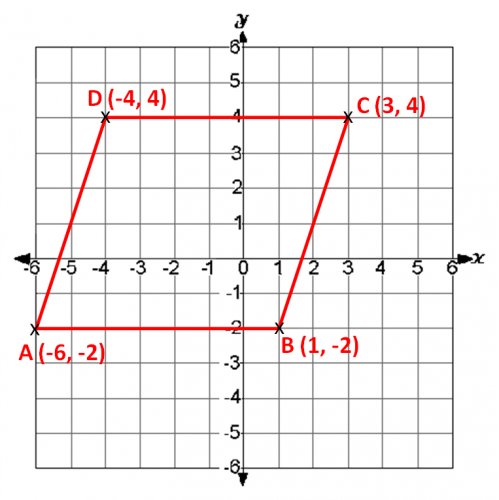# Coordinate Grids (2)

In this worksheet, students must identify the points with the given coordinates on a 4-quadrant coordinate grid.Key stage:  KS 2

Curriculum topic:   Geometry: Position and Direction

Curriculum subtopic:   Use Coordinate Grids

Difficulty level:### QUESTION 1 of 10

We have learned how to read and plot coordinates on a coordinate grid with positive numbers.

Now we extend the grid in all directions to include negative numbers.

Look carefully at how the axes are numbered.Look at the point A.

Its x-coordinate is -5 on the x-axis.

Its y-coordinate is -3 on the y-axis.

Remember the x number comes before the y number.

A has coordinates (-5, -3).

Look at the coordinates of all four points of the parallelogram, shown below.

Remember to read the x before the y.Look at the coordinate grid below.

Which point has coordinates (1, -3)?D

F

G

H

J

K

L

M

N

P

Look at the coordinate grid below.

Which point has coordinates (1, 4)?D

F

G

H

J

K

L

M

N

P

Look at the coordinate grid below.

Which point has coordinates (4, -4)?D

F

G

H

J

K

L

M

N

P

Look at the coordinate grid below.

Which point has coordinates (-3, 1)?D

F

G

H

J

K

L

M

N

P

Look at the coordinate grid below.

Which point has coordinates (-2, 0)?D

F

G

H

J

K

L

M

N

P

Look at the coordinate grid below.

Which point has coordinates (-4, 2)?D

F

G

H

J

K

L

M

N

P

Look at the coordinate grid below.

Which point has coordinates (0, 2)?D

F

G

H

J

K

L

M

N

P

Look at the coordinate grid below.

Which point has coordinates (-3, -3)?D

F

G

H

J

K

L

M

N

P

Look at the coordinate grid below.

Which point has coordinates (-4, 4)?D

F

G

H

J

K

L

M

N

P

Look at the coordinate grid below.

Which point has coordinates (0, -2)?D

F

G

H

J

K

L

M

N

P

• Question 1

Look at the coordinate grid below.

Which point has coordinates (1, -3)?P
EDDIE SAYS
1 on the x-axis.
-3 on the y-axis.
• Question 2

Look at the coordinate grid below.

Which point has coordinates (1, 4)?K
EDDIE SAYS
1 on the x-axis.
4 on the y-axis.
• Question 3

Look at the coordinate grid below.

Which point has coordinates (4, -4)?G
EDDIE SAYS
4 on the x-axis.
-4 on the y-axis.
• Question 4

Look at the coordinate grid below.

Which point has coordinates (-3, 1)?N
EDDIE SAYS
-3 on the x-axis.
1 on the y-axis.
• Question 5

Look at the coordinate grid below.

Which point has coordinates (-2, 0)?D
EDDIE SAYS
-2 on the x-axis.
0 on the y-axis.
• Question 6

Look at the coordinate grid below.

Which point has coordinates (-4, 2)?M
EDDIE SAYS
-4 on the x-axis.
2 on the y-axis.
• Question 7

Look at the coordinate grid below.

Which point has coordinates (0, 2)?J
EDDIE SAYS
0 on the x-axis.
2 on the y-axis.
• Question 8

Look at the coordinate grid below.

Which point has coordinates (-3, -3)?F
EDDIE SAYS
-3 on the x-axis.
-3 on the y-axis.
• Question 9

Look at the coordinate grid below.

Which point has coordinates (-4, 4)?L
EDDIE SAYS
-4 on the x-axis.
4 on the y-axis.
• Question 10

Look at the coordinate grid below.

Which point has coordinates (0, -2)?H
EDDIE SAYS
0 on the x-axis.
-2 on the y-axis.
---- OR ----

Sign up for a £1 trial so you can track and measure your child's progress on this activity.

### What is EdPlace?

We're your National Curriculum aligned online education content provider helping each child succeed in English, maths and science from year 1 to GCSE. With an EdPlace account you’ll be able to track and measure progress, helping each child achieve their best. We build confidence and attainment by personalising each child’s learning at a level that suits them.

Get started